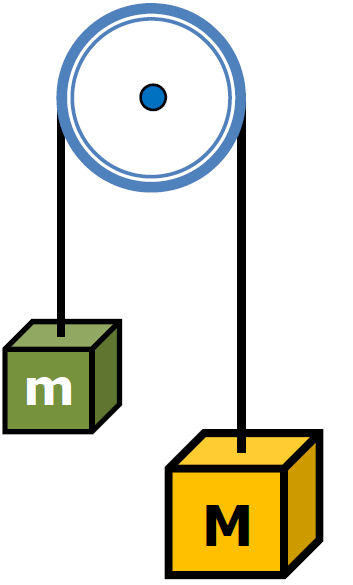﻿ HomeHomeAssuming a frictionless, massless pulley, ...

... determine the a of the block after the large block is released

``
`Let's make clockwise positive`
`Fnet = ma`
` `
`[Mg - mg] = (M + m)a`
` `
``

 g(M - m) = a (m + M)

Only applies when surface frictionless

` `
` `
` `
`Finish This Sentence`

According to Newton's 2nd LAw the acceleration of the 2 masses
comes from weight difference and .....

``
` `
```Force of tension of the rope
on mass m, M?```
``
```Rule - One Rope,
One Tension```
`Let's Make Clockwise Positive`
` `
` `
``
` `
`ΣFy = Ma`
` `
`Mg - Tf = Ma`
` `
`Tf  = Mg - Ma`

`Full Screen`Pulley / Table